Collinearity of 3 points or 3 position vectors

Chapter 10 Class 12 Vector Algebra
Concept wise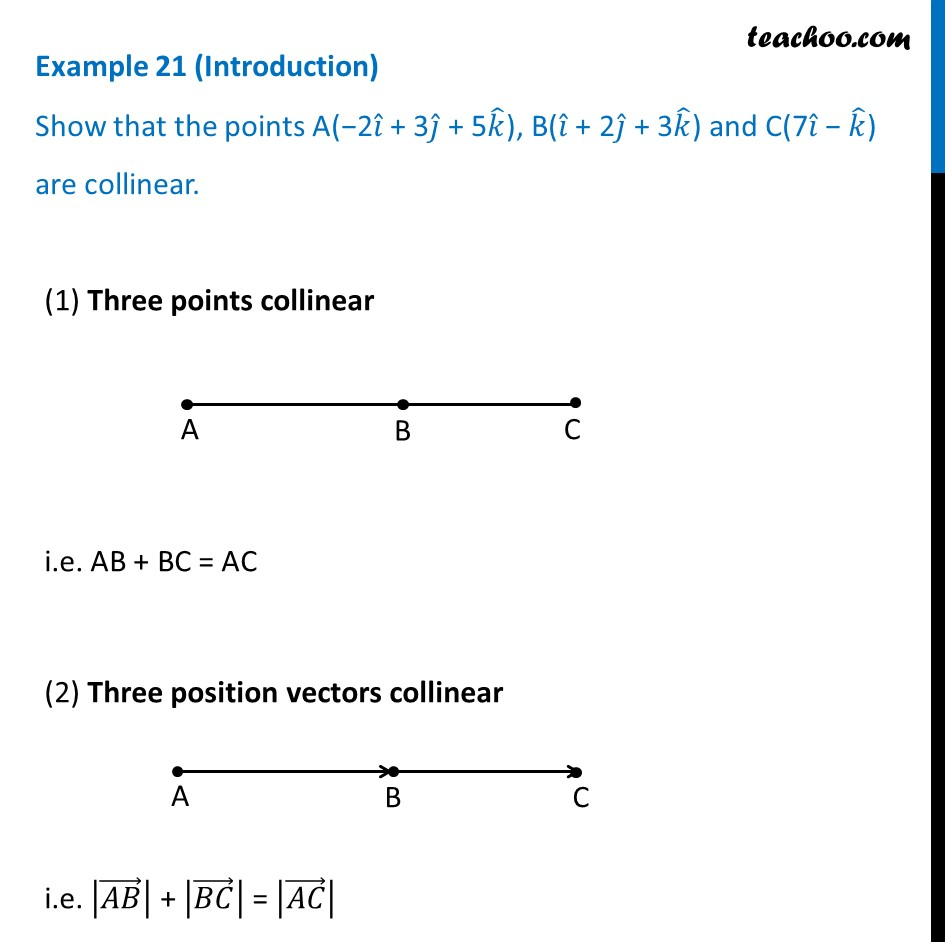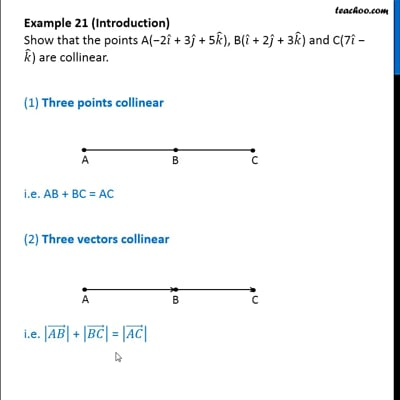This video is only available for Teachoo black users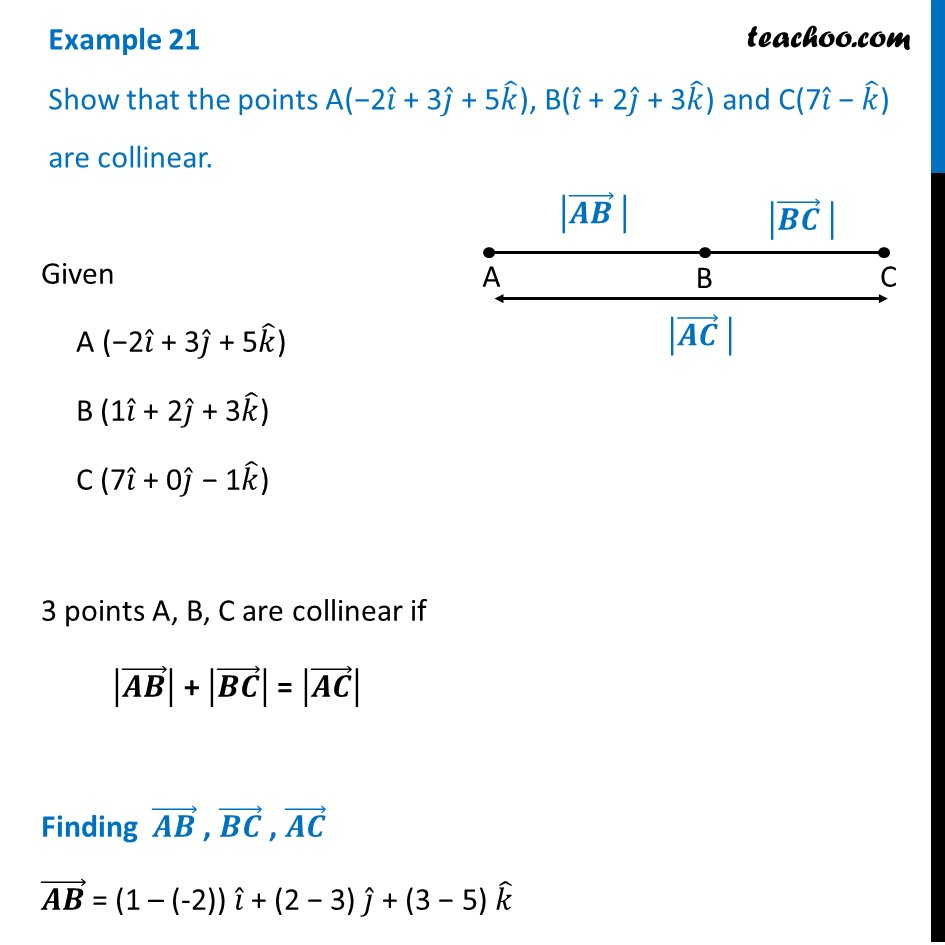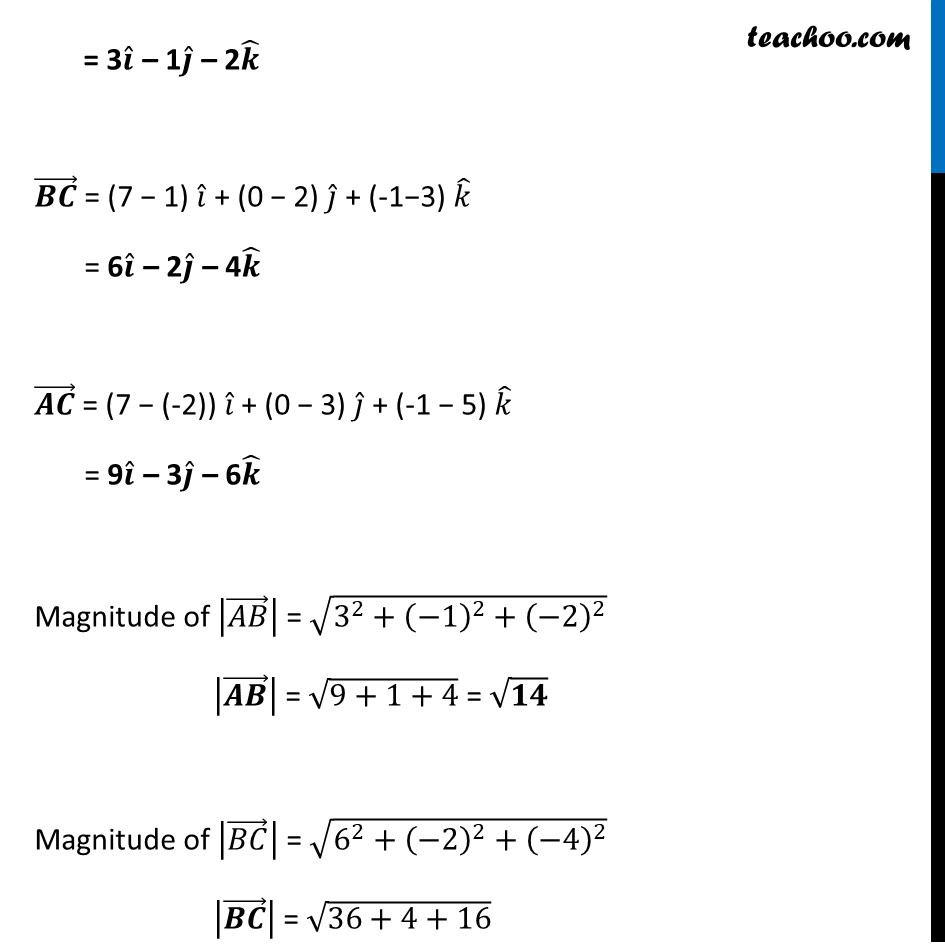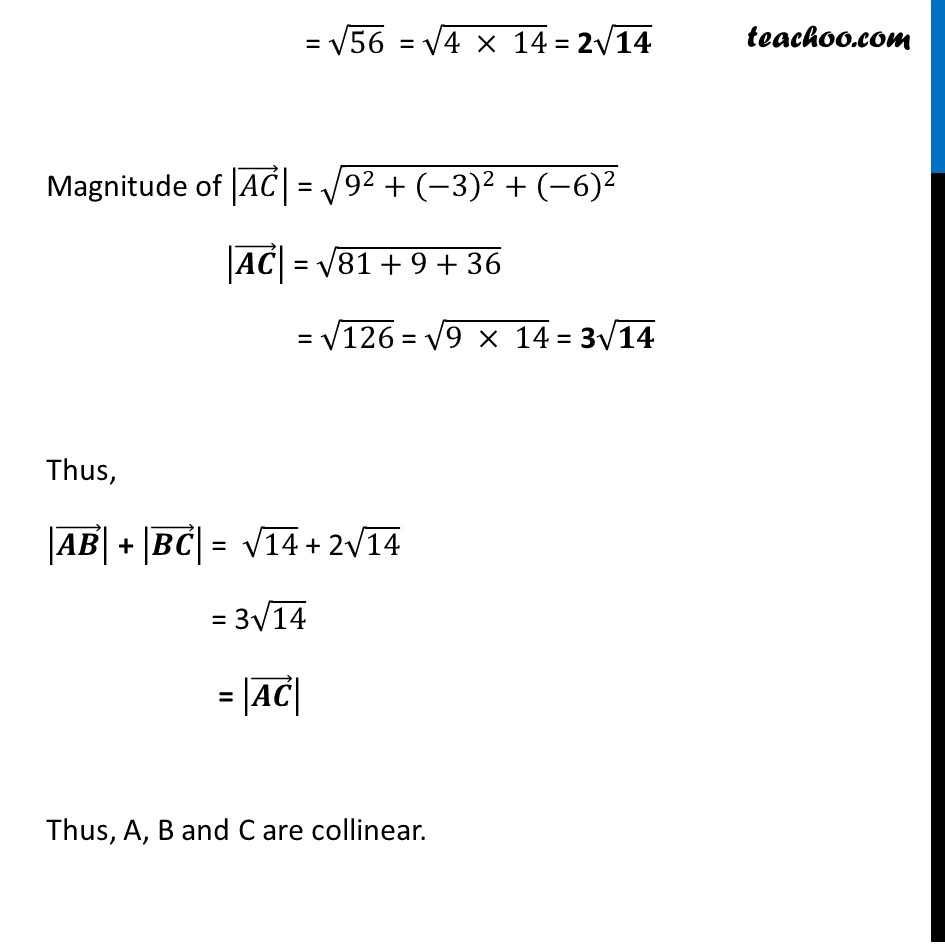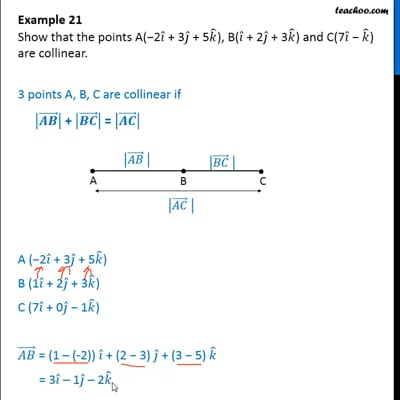This video is only available for Teachoo black users

Introducing your new favourite teacher - Teachoo Black, at only ₹83 per month

### Transcript

Example 21 (Introduction) Show that the points A(−2𝑖 ̂ + 3𝑗 ̂ + 5𝑘 ̂), B(𝑖 ̂ + 2𝑗 ̂ + 3𝑘 ̂) and C(7𝑖 ̂ − 𝑘 ̂) are collinear. (1) Three points collinear i.e. AB + BC = AC (2) Three position vectors collinear i.e. |(𝐴𝐵) ⃗ | + |(𝐵𝐶) ⃗ | = |(𝐴𝐶) ⃗ | Example 21 Show that the points A(−2𝑖 ̂ + 3𝑗 ̂ + 5𝑘 ̂), B(𝑖 ̂ + 2𝑗 ̂ + 3𝑘 ̂) and C(7𝑖 ̂ − 𝑘 ̂) are collinear. Given A (−2𝑖 ̂ + 3𝑗 ̂ + 5𝑘 ̂) B (1𝑖 ̂ + 2𝑗 ̂ + 3𝑘 ̂) C (7𝑖 ̂ + 0𝑗 ̂ − 1𝑘 ̂) 3 points A, B, C are collinear if |(𝑨𝑩) ⃗ | + |(𝑩𝑪) ⃗ | = |(𝑨𝑪) ⃗ | Finding (𝑨𝑩) ⃗ , (𝑩𝑪) ⃗ , (𝑨𝑪) ⃗ (𝑨𝑩) ⃗ = (1 – (-2)) 𝑖 ̂ + (2 − 3) 𝑗 ̂ + (3 − 5) 𝑘 ̂ = 3𝒊 ̂ – 1𝒋 ̂ – 2𝒌 ̂ (𝑩𝑪) ⃗ = (7 − 1) 𝑖 ̂ + (0 − 2) 𝑗 ̂ + (-1−3) 𝑘 ̂ = 6𝒊 ̂ – 2𝒋 ̂ – 4𝒌 ̂ (𝑨𝑪) ⃗ = (7 − (-2)) 𝑖 ̂ + (0 − 3) 𝑗 ̂ + (-1 − 5) 𝑘 ̂ = 9𝒊 ̂ – 3𝒋 ̂ – 6𝒌 ̂ Magnitude of |(𝐴𝐵) ⃗ | = √(3^2+(−1)^2+(−2)^2 ) |(𝑨𝑩) ⃗ | = √(9+1+4) = √𝟏𝟒 Magnitude of |(𝐵𝐶) ⃗ | = √(6^2+(−2)^2+(−4)^2 ) |(𝑩𝑪) ⃗ | = √(36+4+16) = √56 = √(4 × 14) = 2√𝟏𝟒 Magnitude of |(𝐴𝐶) ⃗ | = √(9^2+(−3)^2+(−6)^2 ) |(𝑨𝑪) ⃗ | = √(81+9+36) = √126 = √(9 × 14) = 3√𝟏𝟒 Thus, |(𝑨𝑩) ⃗ | + |(𝑩𝑪) ⃗ | = √14 + 2√14 = 3√14 = |(𝑨𝑪) ⃗ | Thus, A, B and C are collinear.